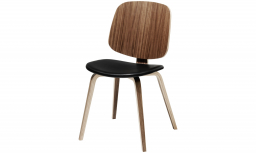# The cost 5

The cost of three chairs and four tables is 320.00. If two chairs and five of the same kind cost 330.00, find the cost of
(i) 4 chairs
(ii) 3 tables

x =  160
y =  150

### Step-by-step explanation:Did you find an error or inaccuracy? Feel free to write us. Thank you!

Tips for related online calculators
Do you have a linear equation or system of equations and looking for its solution? Or do you have a quadratic equation?